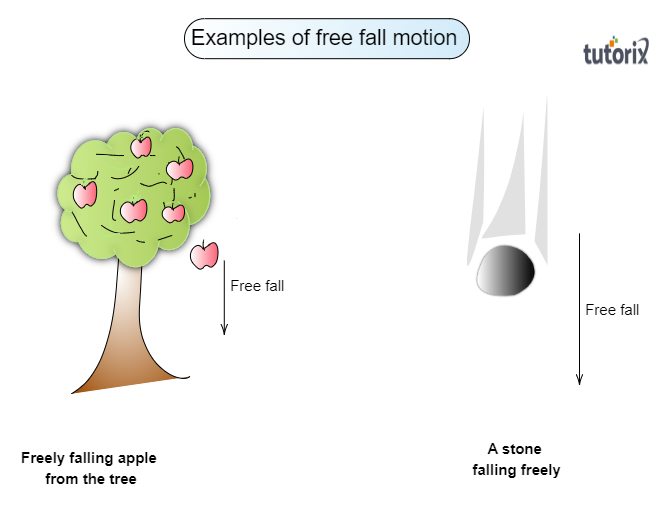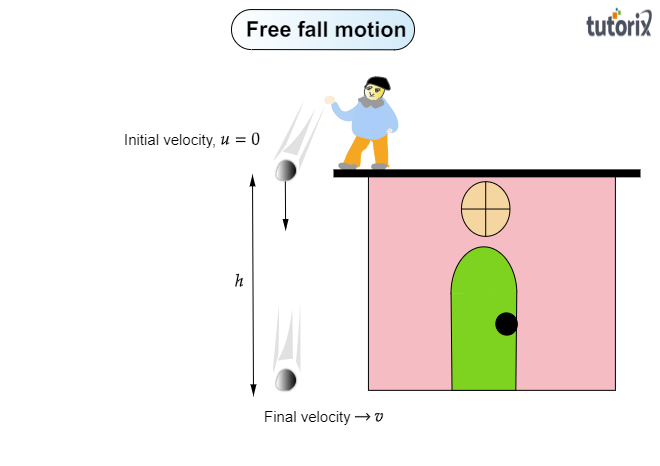# What is the acceleration of free fall?

To get to know the acceleration of free fall, let us know a little about a free fall motion:

Free fall motion:

When a body or object is dropped in the air from a certain height, it begins to fall under the influence of gravity. This motion of the object or body is called a free fall motion and the object is called a freely falling object. Only gravitational force$(gravity)$ acts on the body that attracts it vertically downward to the earth. No other force acts on such a freely falling object.

Examples of free fall:

A fruit falling from the tree after getting ripped and a stone dropped from a certain height are examples of freely falling objects.Acceleration of free fall:

Acceleration of free fall is the acceleration experienced by a freely falling object falling due to gravity.  All objects freely fall at the same rate of acceleration regardless of their mass. Acceleration of free fall is also known as acceleration due to gravity gravitational acceleration. The acceleration of free fall is denoted by $'g'$.

Formula for the acceleration of free fall

When an object is dropped for free fall from a certain height $h$ and it takes $t$ time to reach the ground,Its initial velocity $u=0$

Here, acceleration $a=g$,

On substituting the above values in the three equations of motion, we have:

$v=gt$

$h=\frac{1}{2}gt^2$

$v^2=2gh$

Here, $v$ is the final velocity of the free-falling object when it reaches the ground.

SI unit of the gravitational acceleration is $meter/secon^2$ or $m/s^2$.

On earth, the value of the acceleration of free fall is constant and equal to $9.8\ m/s^2$.